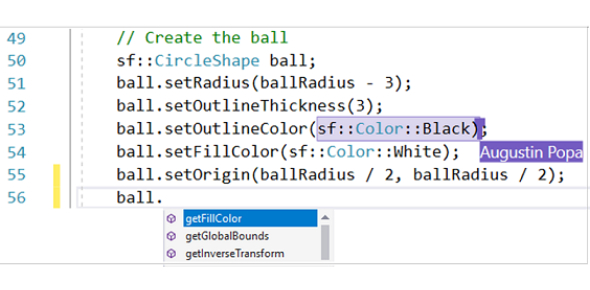# C++ Online Trivia Quiz

15 Questions | Total Attempts: 2092Settings.

• 1.
What is the correct value to return to the operating system upon the successful completion of a program?
• A.

0

• B.

1

• C.

2

• D.

Programs do not return a value.

• E.

-1

• 2.
What is the only function all C++ programs must contain?
• 3.
What symbol is used to state the beginning and the end of blocks of code ?
• A.

( and )

• B.

{ and }

• C.

< and >

• 4.
What symbol punctuation ends almost all lines of C++ code?
• 5.
Which of the following answers is the correct operator to compare two variables
• A.

=

• B.

==

• C.

All of the above

• 6.
Which of the following is true?
• A.

1

• B.

66

• C.

.1

• D.

45

• E.

All of the above

• 7.
Evaluate !(1 && !(0 || 1)).
• A.

True

• B.

False

• 8.
Which of the following shows the correct syntax for an if statement?
• A.

If {expression}

• B.

If (expression)

• C.

If expression

• D.

None of the above

• E.

All of the above

• 9.
Which of the following is the boolean operator for logical-and?
• A.

&

• B.

&&

• C.

|

• D.

None of the above

• 10.
How do you say not equal to?
• A.

=

• B.

==

• C.

• D.

!=

• E.

-=

• 11.
1: #include <iostream.h> 2: int main() 3: { 4: int x = 5; 5: int y = 7; 6: cout "\n"; 7: cout << x + y << " " << x * y; 8: cout "\n"; 9:return 0; 10: } Will Print What
• 12.
{ is called a bracket
• A.

True

• B.

False

• 13.
What simble is used for not in c++
• A.

N

• B.

^

• C.

!

• D.

*

• E.

/*

• 14.
What is the boolan operator for "or"
• 15.
Write a program that will print "Hello World"
Related TopicsBack to top